Question

# pter 4-6 0 Saved Help Save At 25°C, the following heats of reaction are known: 2C1F(g)...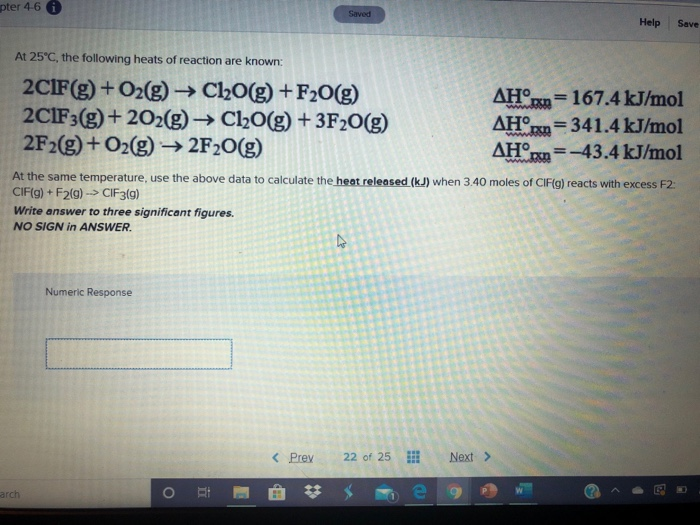pter 4-6 0 Saved Help Save At 25°C, the following heats of reaction are known: 2C1F(g) + O2(g) → Cl2O(g) +F20(g) 2C1F3(g) + 2O2(g) + Cl2O(g) + 3F2O(g) 2F2(g) + O2(g) → 2F20(g) AHO/= 167.4 kJ/mol AHºrn = 341.4 kJ/mol AHºrn=-43.4 kJ/mol At the same temperature, use the above data to calculate the heat released (kJ) when 3.40 moles of CIF(g) reacts with excess F2. CIF(g) + F2(9) --> CIF3(9) Write answer to three significant figures. NO SIGN in ANSWER. Numeric Response < Prey 22 of 25 Next > arch

Given data,

2 ClF (g) + O2 (g) ------> Cl2O (g) + F2O (g)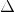H1 = 167.4 kJ/mol ----------- (1)

2 ClF3 (g) + 2 O2 (g) ----------> Cl2O (g) + 3 F2O (g) ,H2 = 341.4 kJ/mol ---------- (2)

2 F2 (g) + O2 (g) ----> 2 F2O (g)H3 = - 43.4 kJ/mol ----------- (3)

Required equation is,

ClF (g) + F2 (g) ------------> ClF3 (g)H = ?

To get the required equation, first, do (1) + (3) - (2)

2 ClF (g) + O2 (g) ------> Cl2O (g) + F2O (g)H1 = 167.4 kJ/mol ----------- (1)

(+)

2 F2 (g) + O2 (g) ----> 2 F2O (g)H3 = - 43.4 kJ/mol ----------- (3)

( - )

2 ClF3 (g) + 2 O2 (g) ----------> Cl2O (g) + 3 F2O (g) ,H2 = 341.4 kJ/mol ---------- (2)

--------------------------------------------------------------------------------------------------------------------------------

2 ClF (g) + 2 F2 (g) ------------> 2 ClF3 (g)H4 = ?

---------------------------------------------------------------------------------------------------------------------------------

Therefore,H4 =H1 +H3 -H2H4 = 167.4 + ( - 43.4 ) - 341.4H4 = - 217.4

This is the heat released when 2 mol of ClF reacts with excess F2.

Then, heat released when 3.40 moles of ClF reacts with excess F2 is,H =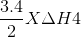H =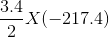H = - 370 kJ

#### Earn Coins

Coins can be redeemed for fabulous gifts.

Similar Homework Help Questions
• ### At 25°C, the following heats of reaction are known: 2CIF(g) + O2(g) → Cl2O(g) + F2O(g)...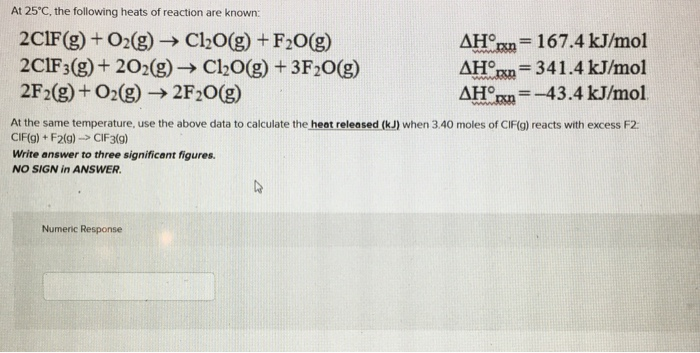At 25°C, the following heats of reaction are known: 2CIF(g) + O2(g) → Cl2O(g) + F2O(g) AHºrn = 167.4 kJ/mol 2C1F3(g) + 202(g) + Cl2O(g) + 3F2O(g) AHºrn = 341.4 kJ/mol 2F2(g) + O2(g) → 2F20(g) AHºrxn=-43.4 kJ/mol At the same temperature, use the above data to calculate the heat released (kJ) when 3.40 moles of CIF(g) reacts with excess F2. CIF(g) + F219) -> CIF3(g) Write answer to three significant figures. NO SIGN in ANSWER. Numeric Response

• ### 9. Given the following data: 2C1F(g) + O2(g) → Cl2O(g) + F20(g) 2C1F3(g) + 2O2(g) → Cl2O(g) + 3F20 2F2(g) + O2(g)...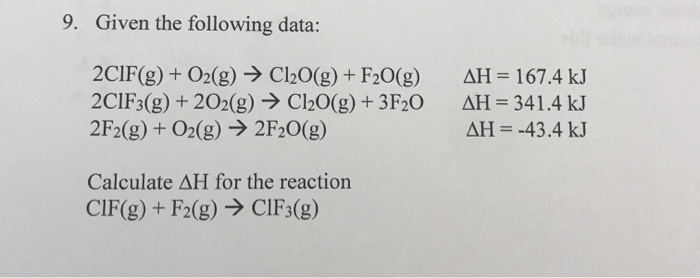9. Given the following data: 2C1F(g) + O2(g) → Cl2O(g) + F20(g) 2C1F3(g) + 2O2(g) → Cl2O(g) + 3F20 2F2(g) + O2(g) → 2F20(g) AH = 167.4 kJ AH = 341.4 kJ AH = -43.4 kJ Calculate AH for the reaction CIF(g) + F2(g) → CIF3(g) 10. Calculate AHº for the following reaction using the AHⓇ information below. 4NH3(g) + 5O2(g) → 4NO(g) + 6H2O(g) AH NH3(g) is -80 kJ/mol AH° NO(g) is 90 kJ/mol AH®, H2O(g) is -242 kJ/mol

• ### At 25°C, the following heats of reaction are known: 2CIF(g) + O2(g) → Cl20(g) + F2。dH'm...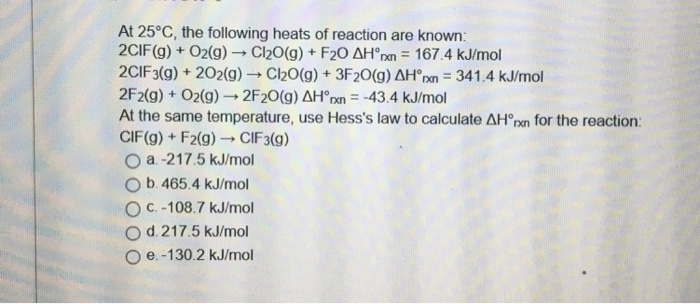At 25°C, the following heats of reaction are known: 2CIF(g) + O2(g) → Cl20(g) + F2。dH'm = 167.4 kJ/mol 2ClF3(g) + 202(g) → Cl20(g) + 3F20(g) ΔH'm-34 1.4 kJ/mol 2F2(g) + O2(g) → 2F20(g) ΔHoxn--43.4 kJ/mol At the same temperature, use Hess's law to calculate ΔHorn for the reaction: CIF(g) + F2(g) → ClF3(g) 0 a-21 7.5 kJ/mol O b 465.4 kJ/mol O c. -108.7 kJ/mol d 217.5 kJ/mol O e-130.2 kJ/mol

• ### At 25°C, the following heats of reaction are known: AH (kJ/mol 167.4 2CIF + 02 →Cl20...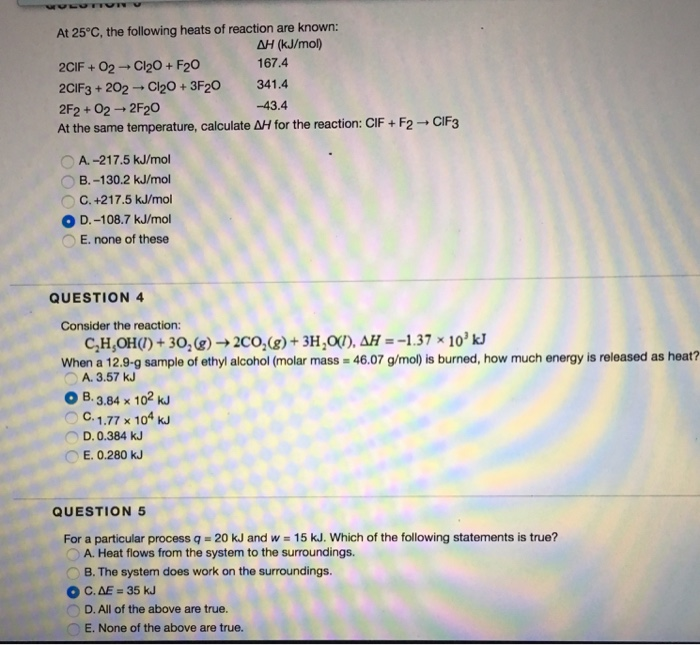At 25°C, the following heats of reaction are known: AH (kJ/mol 167.4 2CIF + 02 →Cl20 + F20 2ClF3 + 202 →Cl20+3F20 341.4 2F2 + 02 → 2F20 At the same temperature, calculate ΔH for the reaction: ClF + F2 → CIF3 -43.4 A. -217.5 kJ/mol B.-130.2 kJ/mol C. +217.5 kJ/mol ○ D.-108.7 kJ/mol E. none of these QUESTION 4 Consider the reaction: When a 12.9-g sample of ethyl alcohol(molar mass 46.07 g/mol) is burned, how much energy is released...

• ### AH° = ? 3.(5pts) Consider the thermochemical reaction: CIF(g) + F (g) ----> CIF3(g) Use the...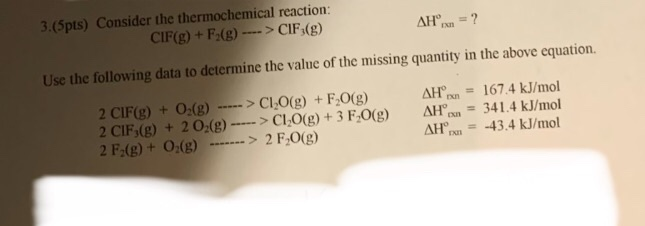AH° = ? 3.(5pts) Consider the thermochemical reaction: CIF(g) + F (g) ----> CIF3(g) Use the following data to determine the value of the missing quantity in the above equation. 2 CIF(g) + O2(g) -----> CI2O(g) + F2O(g) 2 CIF,(g) + 2 O2(g) ----> CI-O(g) + 3 F2O(g) 2 F2(g) + O2(g) -- -> 2 F.0(g) AH AH AH = 167.4 kJ/mol = 341.4 kJ/mol = -43.4 kJ/mol

• ### 3.(5pts)) Consider the thermochemical reaction: 3 CIF(g) + 3F.(g) ---->3 CIF,(g) AHºrn = ? Use the...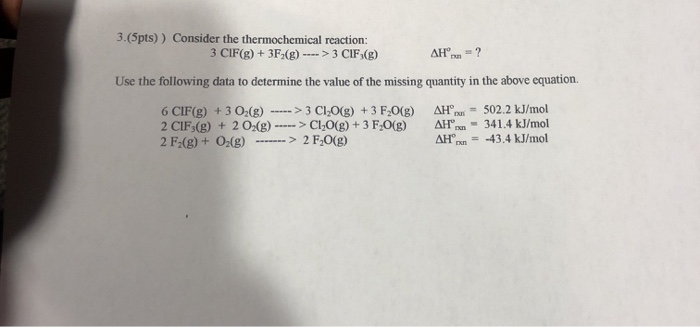3.(5pts)) Consider the thermochemical reaction: 3 CIF(g) + 3F.(g) ---->3 CIF,(g) AHºrn = ? Use the following data to determine the value of the missing quantity in the above equation. 6 CIF(g) + 3 O2(g) ----->3 ClO(g) + 3 F 0(g) 2 CIF3(g) + 2 02(8) -----> CLO(g) + 3 F.0() 2 F2(g) + O2(g) -------> 2 F.O(g) AH AH° AH - 502.2 kJ/mol 341.4 kJ/mol = -43.4 kJ/mol

• ### At 25 degree C, the following heats of reaction are known: 2CIF (g) + O_2 (g)...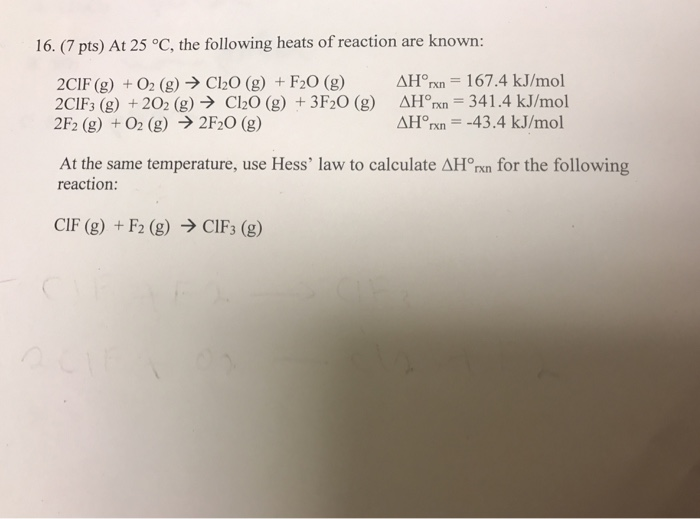At 25 degree C, the following heats of reaction are known: 2CIF (g) + O_2 (g) rightarrow Cl_2O (g)+ F_2O (g) Delta H_rxn^degree = 167.4 kJ/mol 2ClF_3 (g) +2O_2 (g) rightarrow Cl_2O (g) + 3F_2O (g) Delta H_rxn^degree = 341.4 kJ/mol 2F_2 (g) + O_2 (g) rightarrow 2F_2O (g) Delta H_rxn^degree = -43.4 kJ/mol At the same temperature, use Hess' law to calculate Delta H_rxn^degree for the following reaction: ClF (g) + F_2 (g) rightarrow ClF_3 (g)